Axisymmetric Configurations (Steady-State Structures)

Equilibrium, axisymmetric structures are obtained by searching for time-independent, steady-state solutions to the identified set of simplified governing equations.

 |   Tiled Menu   |   Tables of Content   |  Banner Video   |  Tohline Home Page   |

Cylindrical Coordinate Base

We begin by writing each governing equation in Eulerian form and setting all partial time-derivatives to zero:

Equation of Continuity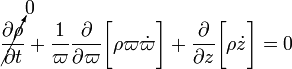$\cancelto{0}{\frac{\partial\rho}{\partial t}} + \frac{1}{\varpi} \frac{\partial}{\partial\varpi} \biggl[ \rho \varpi \dot\varpi \biggr] + \frac{\partial}{\partial z} \biggl[ \rho \dot{z} \biggr] = 0$

The Two Relevant Components of the
Euler Equation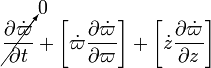$~ \cancelto{0}{\frac{\partial \dot\varpi}{\partial t}} + \biggl[ \dot\varpi \frac{\partial \dot\varpi}{\partial\varpi} \biggr] + \biggl[ \dot{z} \frac{\partial \dot\varpi}{\partial z} \biggr]$$~=$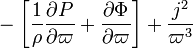$~ - \biggl[ \frac{1}{\rho}\frac{\partial P}{\partial\varpi} + \frac{\partial \Phi}{\partial\varpi}\biggr] + \frac{j^2}{\varpi^3}$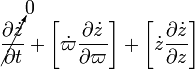$~ \cancelto{0}{\frac{\partial \dot{z}}{\partial t}} + \biggl[ \dot\varpi \frac{\partial \dot{z}}{\partial\varpi} \biggr] + \biggl[ \dot{z} \frac{\partial \dot{z}}{\partial z} \biggr]$$~=$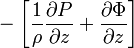$~ - \biggl[ \frac{1}{\rho}\frac{\partial P}{\partial z} + \frac{\partial \Phi}{\partial z} \biggr]$

Adiabatic Form of the
First Law of Thermodynamics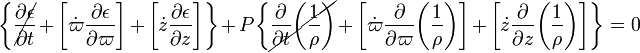$~ \biggl\{\cancel{\frac{\partial \epsilon}{\partial t}} + \biggl[ \dot\varpi \frac{\partial \epsilon}{\partial\varpi} \biggr] + \biggl[ \dot{z} \frac{\partial \epsilon}{\partial z} \biggr]\biggr\} + P \biggl\{\cancel{\frac{\partial }{\partial t}\biggl(\frac{1}{\rho}\biggr)} + \biggl[ \dot\varpi \frac{\partial }{\partial\varpi}\biggl(\frac{1}{\rho}\biggr) \biggr] + \biggl[ \dot{z} \frac{\partial }{\partial z}\biggl(\frac{1}{\rho}\biggr) \biggr] \biggr\} = 0$

Poisson Equation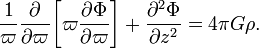$\frac{1}{\varpi} \frac{\partial }{\partial\varpi} \biggl[ \varpi \frac{\partial \Phi}{\partial\varpi} \biggr] + \frac{\partial^2 \Phi}{\partial z^2} = 4\pi G \rho .$

The steady-state flow field that will be adopted to satisfy both an axisymmetric geometry and the time-independent constraint is,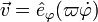$~\vec{v} = \hat{e}_\varphi (\varpi \dot\varphi)$. That is,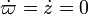$~\dot\varpi = \dot{z} = 0$ but, in general,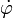$~\dot\varphi$ is not zero and can be an arbitrary function of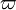$~\varpi$ and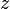$~z$, that is,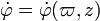$~\dot\varphi = \dot\varphi(\varpi,z)$. We will seek solutions to the above set of coupled equations for various chosen spatial distributions of the angular velocity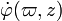$~\dot\varphi(\varpi,z)$, or of the specific angular momentum,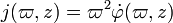$~j(\varpi,z) = \varpi^2 \dot\varphi(\varpi,z)$.

After setting the radial and vertical velocities to zero, we see that the 1st (continuity) and 4th (first law of thermodynamics) equations are trivially satisfied while the 2nd & 3rd (Euler) and 5th (Poisson) give, respectively,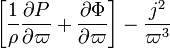$~ \biggl[ \frac{1}{\rho}\frac{\partial P}{\partial\varpi} + \frac{\partial \Phi}{\partial\varpi}\biggr] - \frac{j^2}{\varpi^3}$$~=$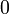$~0$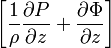$~ \biggl[ \frac{1}{\rho}\frac{\partial P}{\partial z} + \frac{\partial \Phi}{\partial z} \biggr]$$~=$$~0$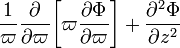$~ \frac{1}{\varpi} \frac{\partial }{\partial\varpi} \biggl[ \varpi \frac{\partial \Phi}{\partial\varpi} \biggr] + \frac{\partial^2 \Phi}{\partial z^2}$$~=$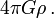$~4\pi G \rho \, .$

As has been outlined in our discussion of supplemental relations for time-independent problems, in the context of this H_Book we will close this set of equations by specifying a structural, barotropic relationship between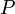$~P$ and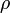$~\rho$.

Spherical Coordinate Base

Equation of Continuity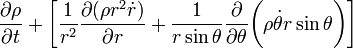$~ \frac{\partial \rho}{\partial t} + \biggl[ \frac{1}{r^2} \frac{\partial (\rho r^2 \dot{r})}{\partial r} + \frac{1}{r\sin\theta} \frac{\partial }{\partial\theta} \biggl( \rho \dot\theta r \sin\theta \biggr) \biggr]$$~=$$~0$

The Two Relevant Components of the
Euler Equation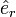$~{\hat{e}}_r$: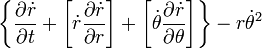$\biggl\{ \frac{\partial \dot{r}}{\partial t} + \biggl[ \dot{r} \frac{\partial \dot{r}}{\partial r} \biggr] + \biggl[ \dot\theta \frac{\partial \dot{r}}{\partial\theta} \biggr] \biggr\} - r {\dot\theta}^2$ =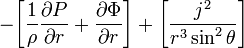$- \biggl[ \frac{1}{\rho} \frac{\partial P}{\partial r}+ \frac{\partial \Phi }{\partial r} \biggr] + \biggl[ \frac{j^2}{r^3 \sin^2\theta} \biggr]$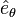$~{\hat{e}}_\theta$: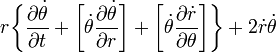$r \biggl\{ \frac{\partial \dot{\theta}}{\partial t} + \biggl[ \dot{\theta} \frac{\partial \dot{\theta}}{\partial r} \biggr] + \biggl[ \dot\theta \frac{\partial \dot{r}}{\partial\theta} \biggr] \biggr\} + 2\dot{r} \dot\theta$ =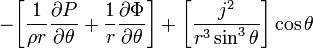$- \biggl[ \frac{1}{\rho r} \frac{\partial P}{\partial\theta} + \frac{1}{r} \frac{\partial \Phi}{\partial\theta} \biggr] + \biggl[ \frac{j^2}{r^3 \sin^3\theta} \biggr] \cos\theta$

Adiabatic Form of the
First Law of Thermodynamics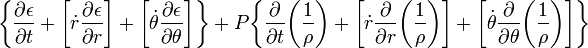$~ \biggl\{ \frac{\partial \epsilon}{\partial t} + \biggl[ \dot{r} \frac{\partial \epsilon}{\partial r} \biggr] + \biggl[ \dot\theta \frac{\partial \epsilon}{\partial\theta} \biggr] \biggr\} + P\biggl\{ \frac{\partial }{\partial t} \biggl( \frac{1}{\rho}\biggr) + \biggl[ \dot{r} \frac{\partial }{\partial r} \biggl( \frac{1}{\rho}\biggr) \biggr] + \biggl[ \dot\theta \frac{\partial }{\partial\theta} \biggl( \frac{1}{\rho}\biggr) \biggr] \biggr\}$$~=$$~0$

Poisson Equation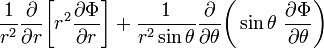$~ \frac{1}{r^2} \frac{\partial }{\partial r} \biggl[ r^2 \frac{\partial \Phi }{\partial r} \biggr] + \frac{1}{r^2 \sin\theta} \frac{\partial }{\partial \theta}\biggl(\sin\theta ~ \frac{\partial \Phi}{\partial\theta}\biggr)$$~=$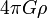$~4\pi G\rho$

where the pair of "relevant" components of the Euler equation have been written in terms of the specific angular momentum,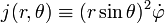$~j(r,\theta) \equiv (r\sin\theta)^2 \dot\varphi$,

which is a conserved quantity in axisymmetric systems.

Given that our aim is to construct steady-state configurations, we should set the partial time-derivative of all scalar quantities to zero; in addition, we will assume that both meridional-plane velocity components,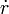$\dot{r}$ and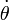$~\dot{\theta}$, to zero — initially as well as for all time. As a result of these imposed conditions, both the equation of continuity and the first law of thermodynamics are automatically satisfied; the Poisson equation remains unchanged; and the left-hand-sides of the pair of relevant components of the Euler equation go to zero. The governing relations then take the following, considerably simplified form:

Spherical Coordinate Base

Poisson Equation$~ \frac{1}{r^2} \frac{\partial }{\partial r} \biggl[ r^2 \frac{\partial \Phi }{\partial r} \biggr] + \frac{1}{r^2 \sin\theta} \frac{\partial }{\partial \theta}\biggl(\sin\theta ~ \frac{\partial \Phi}{\partial\theta}\biggr)$$~=$$~4\pi G\rho$

The Two Relevant Components of the
Euler Equation$~{\hat{e}}_r$:$~0$ =$- \biggl[ \frac{1}{\rho} \frac{\partial P}{\partial r}+ \frac{\partial \Phi }{\partial r} \biggr] + \biggl[ \frac{j^2}{r^3 \sin^2\theta} \biggr]$$~{\hat{e}}_\theta$:$~0$ =$- \biggl[ \frac{1}{\rho r} \frac{\partial P}{\partial\theta} + \frac{1}{r} \frac{\partial \Phi}{\partial\theta} \biggr] + \biggl[ \frac{j^2}{r^3 \sin^3\theta} \biggr] \cos\theta$